# Class 12 Maths NCERT Solutions for Chapter 4 Determinants Exercise 4.6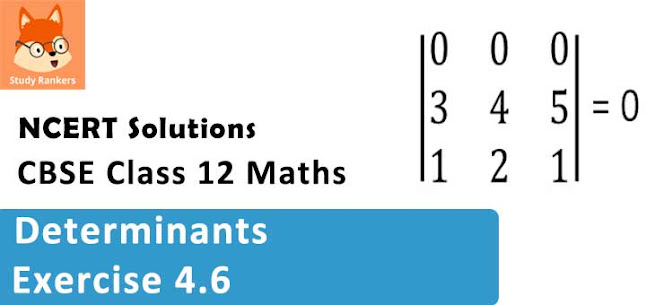### Determinants Exercise 4.6 Solutions

1. Examine the consistency of the system of equations.
+ 2= 2
2x + 3= 3

Solution

The given system of equation is :
x + 2y = 2
2x + 3y = 3
The given system of equations can be written in the form of AX = B, where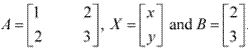Now,
|A| = 1(3) - 2(2) = 3 - 4 = -1 ≠ 0
∴ A is non - singular.
Therefore, A-1 exists.
Hence, the given system of equations is consistent.

2. Examine the consistency of the system of equations.
2− y = 5
x + = 4

Solution

The given system of equations is :
2x - y = 5
x + y = 4
The given system of equations can be written in the form of AX = B, where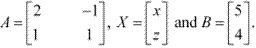Now,
|A| = 2|1| - (-1)(1) = 2 + 1 = 3 ≠ 0
∴ A is non - singular.
Therefore, A-1 exists.
Hence, the given system of equations is consistent.

3. Examine the consistency of the system of equations.
x + 3y = 5
2x + 6y = 8

Solution

The given system of equations is :
x + 3y = 5
2x + 6y = 8
The given system of equations can be written in the form of AX = B, where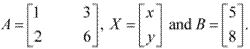Now,
|A| = 1(6) - 3(2) = 6 - 6 = 0
∴ A is a singular matrix.
Now,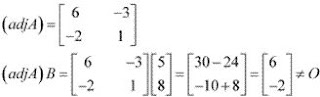Thus, the solution of the given system of equations does not exist. Hence, the system of equations is inconsistent.

4. Examine the consistency of the system of equations.
x + y z = 1
2x + 3y + 2z = 2
ax + ay + 2az = 4

Solution

The given system of equations is :
x + y + z = 1
2x + 3y + 2z = 2
ax + ay + 2az = 4
This system of equations can be written in the form AX = B, where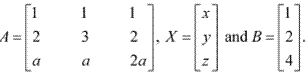Now,
|A| = 1(6a - 2a) - 1(4a - 2a) + 1(2a - 3a)
= 4a - 2a - a = 4a - 3a = a ≠ 0
∴ A is non - singular.
Therefore, A-1 exists.
Hence, the given system of equations is consistent.

5. Examine the consistency of the system of equations.
3x − y − 2z = 2
2y − z = −1
3x − 5y = 3

Solution

The given system of equations is :
3x - y - 2z = 2
2y - z = - 1
3x - 5y = 3
This system of equations can be written in the form of AX = B, where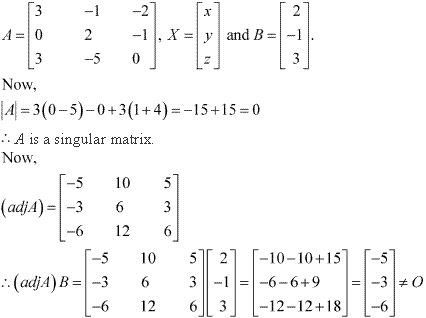Thus, the solution of the given system of equations does not exist. Hence, the system of equations is inconsistent.

6. Examine the consistency of the system of equations.
5x − y + 4z = 5
2x + 3y + 5z = 2
5x − 2y + 6z = −1

Solution

The given system of equations is :
5x − y + 4z = 5
2x + 3y + 5z = 2
5x − 2y + 6z = −1
This system of equations can be written in the form of AX = B, where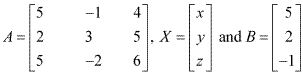Now,
|A| = 5(18 + 10) + 1(12 - 25) + 4(- 4 - 15)
= 5(28) + 1(-13) + 4(-19)
= 140 - 13 - 76
= 51 ≠ 0
A is non  - singular.
Therefore, A-1 exists.
Hence, the given system of equations is consistent.

7. Solve system of linear equations, using matrix method.
5x + 2y = 4
7x + 3y = 5

Solution

The given system of equations can be written in the form of AX = B, where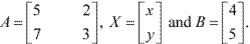Now, |A| = 15 - 14 = 1 ≠ 0.
Thus, A is non - singular. Therefore, its inverse exists.
Now,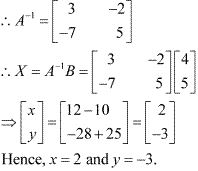8. Solve system of linear equations, using matrix method.
2x – y = –2
3x + 4y = 3

Solution

The given system of equations can be written in the form of AX = B, where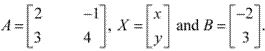Now,
|A| = 8 + 3 = 11 ≠ 0
Thus, A is non - singular. Therefore, its inverse exists.
Now,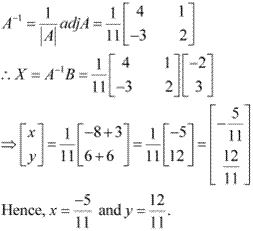9. Solve system of linear equations, using matrix method.
4x – 3y = 3
3x – 5y = 7

Solution

The given system of equation can be written in the form of AX = B, where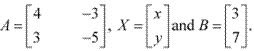Now,
|A| = -20 + 9 = -11≠ 0
Thus,  A is non - singular. Therefore, its inverse exists.
Now,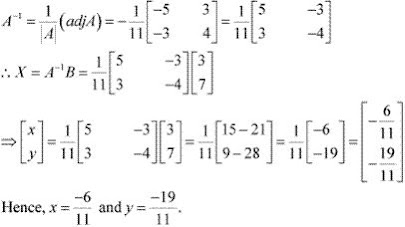10.Solve system of linear equations, using matrix method.
5x + 2y = 3
3x + 2y = 5

Solution

The given system of equations can be written in the form of AX = B, where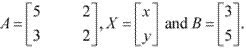Now,
|A| = 10 - 6 = 4 ≠ 0
Thus,  A is non - singular. Therefore, its inverse exists.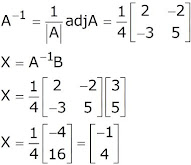11. Solve system of linear equations, using matrix method.
2x + y + z = 1
x – 2y – z = 3/2
3y – 5z = 9

Solution

The given system of equations can be written in the form of AX = B, where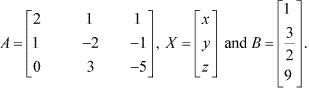Now,
|A| = 2(10 + 3) - 1(-5 - 3) + 0 = 2(13) - 1(-8) = 26 + 8 = 34 ≠ 0
Thus, A is non - singular. Therefore, its inverse exists.
Now,
A11 = 13, A12 = 5, A13 = 3
A21 = 8, A22 = -10 , A23 = -6
A31 = 1, A32 = 3, A33 = -5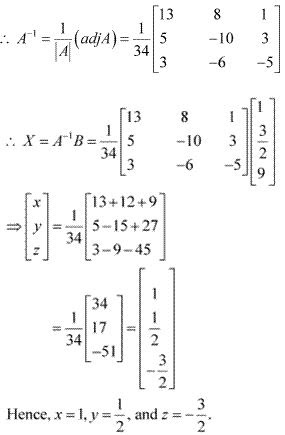12. Solve system of linear equations, using matrix method.
x − y + z = 4
2x + y − 3z = 0
x + y + z = 2

Solution

The given system of equations can be written in the form of AX= B, where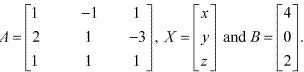Now,
|A| = 1(1 + 3) + 1(2 + 3) + 1(2 - 1) = 4 + 5 + 1= 10 ≠ 0
Thus, A is non - singular. Therefore, its inverse exists.
Now,
A11 = 4, A12 = -5, A13 = 1
A21 = 2, A22 = 0 , A23 = -2
A31 = 2, A32 = 5, A33 = 3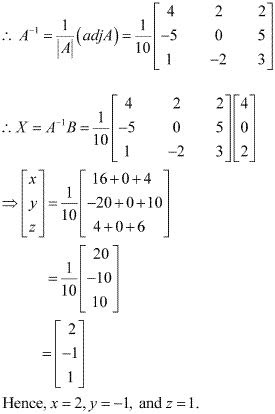13. Solve system of linear equations, using matrix method.
2x + 3y + 3z = 5
x − 2y + z = −4
3x − y − 2z = 3

Solution

The given system of equations can be written in the form AX = B, where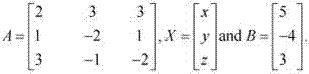Now,
|A| = 2(4 + 1) - 3(-2 - 3) + 3(-1 + 6)
= 2(5) - 3(-5) + 3(5)
= 10 + 15 + 15
= 40 ≠ 0
Thus, A is non - singular. Therefore, its inverse exists.
Now,
A11 = 5, A12 = 5, A13 = 5
A21 = 3, A22 = -13 , A23 = 11
A31 = 9, A32 = 1, A33 = -7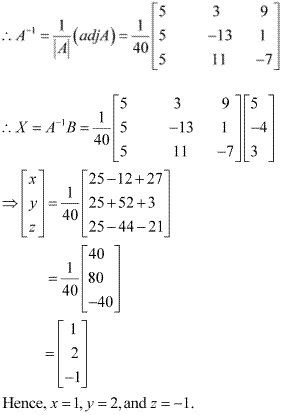14. Solve system of linear equations, using matrix method.
x − y + 2z = 7
3x + 4y − 5z = −5
2x − y + 3z = 12

Solution

The given system of equations can be written in the form of AX = B, where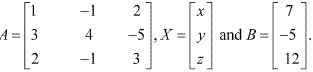Now,
|A| = 1(12 - 5) + 1(9 + 10) + 2(-3 - 8)
= 7 + 19 - 22
= 4 ≠ 0
Thus, A is non - singular. Therefore, its inverse exists.
Now,
A11 = 7, A12 = -19, A13 = -11
A21 = 1, A22 = -1 , A23 = -1
A31 = -3, A32 = 11, A33 = 7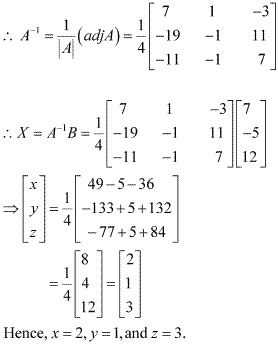15. If A =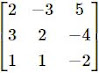find A−1. Using A−1 solve the system of equations
2x – 3y + 5z = 11
3x + 2y – 4z = – 5
x + y – 2z = – 3

Solution

A =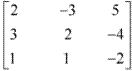∴ |A| = 2(- 4 + 4) + 3(-6 + 4) + 5(3 - 2) = 0 - 6 + 5 = -1 ≠ 0
Now,
A11 = 0, A12 = 2, A13 = 1
A21 = -1, A22 = -9 , A23 = -5
A31 = 2, A32 = 23, A33 = 13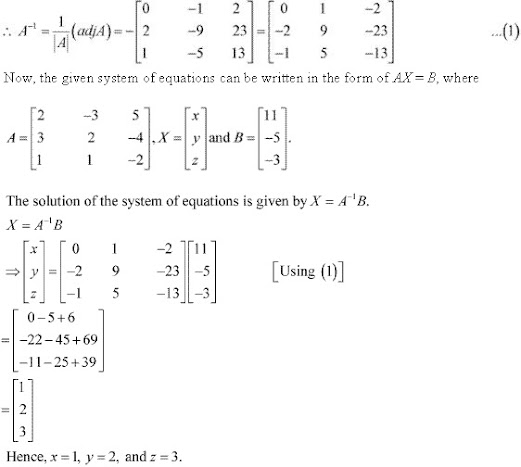16. The cost of 4 kg onion, 3 kg wheat and 2 kg rice is Rs 60. The cost of 2 kg onion, 4 kg wheat and 6 kg rice is Rs 90. The cost of 6 kg onion 2 kg wheat and 3 kg rice is Rs 70. Find cost of each item per kg by matrix method.

Solution

Let the cost of onions, wheat, and rice per kg be Rs x, Rs y, and Rs z respectively.
Then, the given situation can be represented by a system of equations as :
4x +3y + 2z = 60
2x + 4y + 6z = 90
6x + 2y + 3z = 70
This system of equations can be written in the form of AX = B, where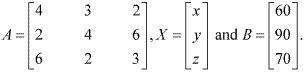|A| = 4(12 - 12) - 3(6 - 36) + 2(4 - 24)
= 0 + 90 - 40 = 50 ≠ 0
Now,
A11 = 0, A12 = 30, A13 = -20
A21 = -5, A22 = 0 , A23 = 10
A31 = 10, A32 = -20, A33 = 10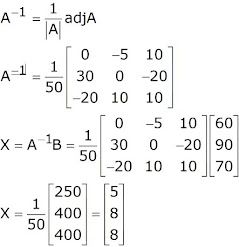x = 5, y = 8 and z = 8
Hence, the cost of onion per kg is Rs. 5, the cost of wheat per kg is Rs.  8 and the cost of rice per kg is Rs. 8.